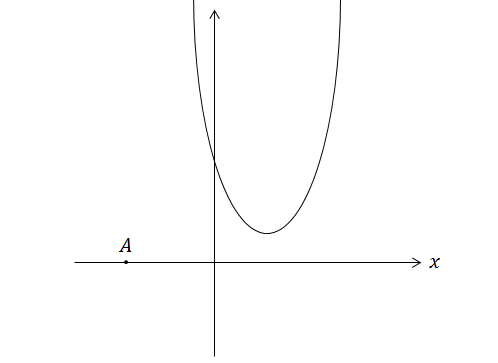Geometry

# Conics - Parabola - General

If the two parabolas $y=2x^2-3x+6$ and $y=-2x^2-ax+1$ meet at a single point, what is the product of all possible values for $a?$

What is the range of $a$ such that the roots of $2x^2+ax+17=0$ are larger than $-17?$Consider a parabola $y=3x^2-5x+2$ and a line that passes through $(-2,0)$ on the coordinate plane. If the parabola and line intersect at exactly two distinct points, what is the possible range for the slope $m$ of the line?

Find the vertex of the parabola $y=4x^2+5x+5.$

A parabola passes through the following three points: $(3,0), (9,0), (1,64).$ What is the vertex of the parabola?

×